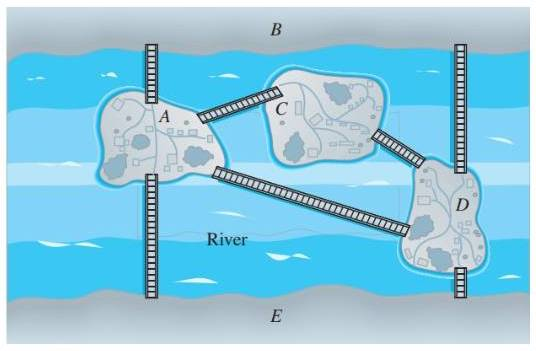Chapter 10.1, Problem 18ES### Discrete Mathematics With Applicat...

5th Edition
EPP + 1 other
ISBN: 9781337694193

#### Solutions

Chapter
Section### Discrete Mathematics With Applicat...

5th Edition
EPP + 1 other
ISBN: 9781337694193
Textbook Problem
1 views

# Is it possible to take a walk around the city whose map is shown below, starting and ending at the same point and crossing each bridge exactly once? If so, how can this be done?To determine

Is it possible to take a walk around the city whose map is shown below, starting and ending at the same point and crossing each bridge exactly once? If so, how can this be done?

Explanation

Given information:

Calculation:

Yes, it is possible.

Expressing the situation graphically.

### Still sussing out bartleby?

Check out a sample textbook solution.

See a sample solution

#### The Solution to Your Study Problems

Bartleby provides explanations to thousands of textbook problems written by our experts, many with advanced degrees!

Get Started